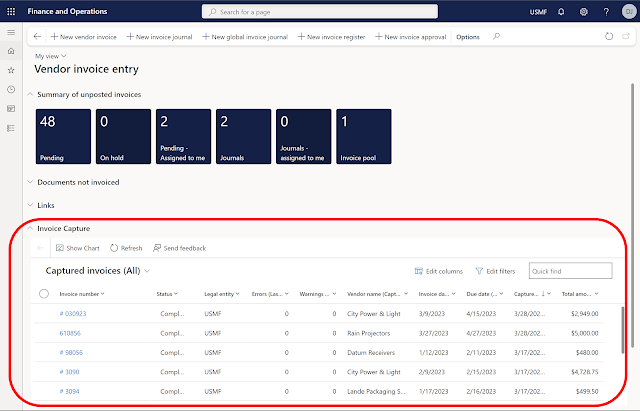### Using Full Page Apps in Dynamics 365 Finance### COVID 19 Dashboard - Article 3 - Adding some key measures - unique time functions

I will continue to build on my earlier posts about my COVID19 Dashboard.  In this series, I am writing a few different blog articles describing not only how I built it, but some things I learned in the process.
The current list of planned topics include:

PART 3 – Adding some key measures

In my earlier posts, I spent time explaining where I acquired the data and some key steps I took in transforming the data into the data model.  In this post, I will explain some of the DAX I used to create some of the key measures for the visualizations.  One of the first steps in design is to identify what questions you are trying to answer or the story you are trying to tell with your visualizations and reports.  In thinking about everything going on with Covid 19, I felt it was not only important to know how many total cases and total deaths, but I wanted to understand how the numbers were changing daily.
• What was the rate of increase?
• Is it increasing faster today than it did yesterday?
• How long did it take for cases to double?
• How long until they double again?

Let’s look at four of the measures that are used in this dashboard.
• Daily increase cases
• Confirmed cases DoD%
• Cases double in
• Cases est. to double again

Daily increase cases – this measure looks at the percent of change between the new cases each day.  This is a percent increase math problem.  Percent Increase = (today’s new cases – yesterday’s new cases) / yesterday’s new cases.  So, to calculated this in DAX, we need today’s cases amount and yesterday’s cases amount.  Since today’s cases will be the maximum date in the data, I used the following DAX formulas to calculate today’s and yesterday’s cases.

First, I crease a Sum Cases measure so I could reference it in other measure.

Sum Cases = sum('US Covid Activity'[Daily Cases])

New Cases Today = CALCULATE([Sum Cases],FILTER(ALL('Date'),'Date'[Date]=MAX('Date'[Date])))

New Cases Yesterday = CALCULATE([Sum Cases],FILTER(ALL('Date'),'Date'[Date]=MAX('Date'[Date])-1))

Once I had the cases I could use a simple DIVIDE function.

Daily Increase Cases = DIVIDE([New Cases Today]-[New Cases Yesterday],[New Cases Yesterday],0)

Confirmed cases DoD% - this measure looks at the day of day percent growth in total cases.  This is also a percent increase math problem.  Percent Increase = (today’s total cases – yesterday’s total cases) / yesterday’s total cases.

In this case, I used a Variable to calculate the Previous Day

Confirmed Cases DoD% =
//Calculate Day of Day % of change
//Set variable for Prior day Life to Date total
VAR __PREV_DAY =
CALCULATE(
SUM('US Covid Activity'[Daily Cases]),
)
//Calculate Net Change in cases (Today LTD less Yesterday LTD)
//Divided by Yesterday LTD
RETURN
DIVIDE(
SUM('US Covid Activity'[Daily Cases]) - __PREV_DAY,
__PREV_DAY
)

Cases Doubles In – This measure looks back in time to see how many days it has taken to double the number of cases.  For example, if today’s total cases are 100,000, then how many has it been since the cases were 50,000.  This seemed to be a complex problem, but fortunately an internet search did not let me down.  I was able to find a close example at https://www.thebiccountant.com/2020/03/22/calculating-doubling-times-with-dax-in-power-bi/  I had to make some slight changes to the example provided to get it to work in my scenario.  Below is the code I used.

Doubled in Days =
//SET THE CURRENT DATE
VAR CURRENTDAY = MAX('US Covid Activity'[Date])
// GET HALF OF TODAYS VALUE
VAR THRESHOLDVALUE =
CALCULATE(SUM('US Covid Activity'[Confirmed Cases]),'US Covid                           Activity'[Date]=CURRENTDAY) / 2
//Get the day when the number of cases was half as much as today
//FILTER returns dates at which the total sum of confirmed cases was below the threshold
VAR DayOfHalf =
CALCULATE(MAXX('US Covid Activity','US Covid
Activity'),'US Covid Activity'[Date]), "AMOUNT",CALCULATE(Sum('US Covid
Activity'[Confirmed Cases]))),[AMOUNT]<=THRESHOLDVALUE))
//Calculated the date difference between today and the date the amount was half
VAR DoublingTime = DATEDIFF(DayOfHalf,CURRENTDAY,DAY)
RETURN
DoublingTime

Cases Est. to Double Again – This measure looks at the confirmed cases day over day % growth that was calculated above, and estimates how many days until the number of cases will double.  This uses the doubling time formula.  I was able to find information on this math formula at https://www.double-entry-bookkeeping.com/number-of-periods/doubling-time-formula/

The formula for doubling time is LN(2)/LN(1 + i).
• The (i) is the rate (confirmed cases DoD%).
• LN returns the natural logarithm of a number.

The DAX formula was

Est. Days to Double = ROUND(DIVIDE(ln(2),ln(1+[Confirmed Cases DoD%])),0)

Note- I used the Round function to round to the nearest whole day.

I also created these measures for the global cases table in my data model.

In my next article, I will look at some additional measures I created around U.S. population.